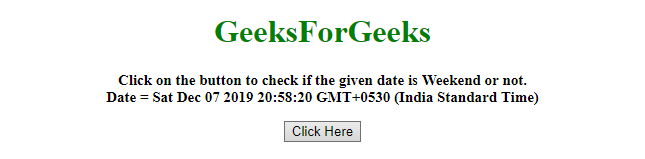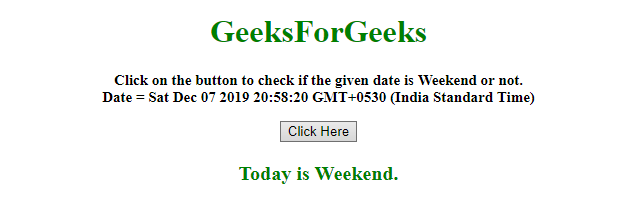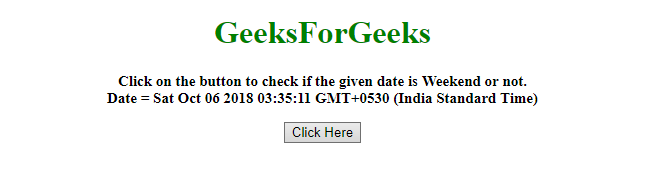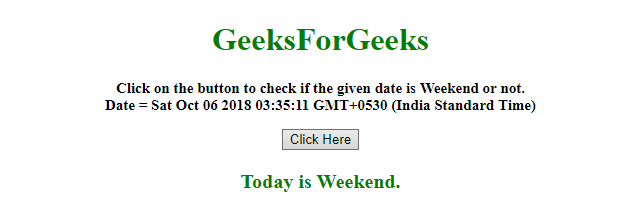Related Articles

# How to check if the given date is weekend ?

• Last Updated : 13 Dec, 2019

Given a date and the task is to determine if the given date is weekend (In this case we are considering Saturday as weekend). There are two methods to solve this problem which are discussed below:

Approach 1:

• Use .getDay() method on the Date object to get the day.
• Check if it is 6 or not (6 denotes Saturday).

Example: This example implements the above approach.

 ` ``<``html``> `` ` `<``head``> ``    ``<``title``> ``        ``How to check if the given``        ``date is weekend ?     ``    ```` `` ` `<``body` `style` `= ``"text-align:center;"``> ``     ` `    ``<``h1` `style` `= ``"color:green;"` `> ``        ``GeeksForGeeks ``    ````     ` `    ``<``p` `id` `= ``"GFG_UP"` `style` `=``        ``"font-size: 15px; font-weight: bold;"``>``    ````     ` `    ``<``button` `onclick` `= ``"gfg_Run()"``> ``        ``Click Here``    ````     ` `    ``<``p` `id` `= ``"GFG_DOWN"` `style = "color:green;``        ``font-size: 20px; font-weight: bold;">``    ````     ` `    ``<``script``>``        ``var el_up = document.getElementById("GFG_UP");``        ``var el_down = document.getElementById("GFG_DOWN");``        ``var date = new Date();``         ` `        ``el_up.innerHTML = "Click on the button to "``                ``+ "check if the given date is Weekend"``                ``+ " or not.<``br``>Date = " + date;``         ` `        ``function gfg_Run() {``            ``var day = date.getDay();``            ``var ans = (day === 6);``             ` `            ``if (ans) {``                ``ans = "Today is Weekend.";``            ``} else {``                ``ans = "Today is not Weekend.";``            ``}``            ``el_down.innerHTML = ans;``        ``} ``    `` `` `` ` ``

Output:

• Before clicking on the button:• After clicking on the button:Approach 2:

• Use .toString() method to convert the Date object to string.
• Get the String from the date object using .substring() method.
• Compare it to the string “Sat” to get the answer.

Example: This example implements the above approach.

 ` ``<``html``> `` ` `<``head``> ``    ``<``title``> ``        ``How to check if the given``        ``date is weekend ?``    ```` `` ` `<``body` `style` `= ``"text-align:center;"``> ``     ` `    ``<``h1` `style` `= ``"color:green;"` `> ``        ``GeeksForGeeks ``    ````     ` `    ``<``p` `id` `= ``"GFG_UP"` `style` `= ``        ``"font-size: 15px; font-weight: bold;"``>``    ````     ` `    ``<``button` `onclick` `= ``"gfg_Run()"``> ``        ``Click Here``    ````     ` `    ``<``p` `id` `= ``"GFG_DOWN"` `style = "color:green;``        ``font-size: 20px; font-weight: bold;">``    ````     ` `    ``<``script``>``        ``var el_up = document.getElementById("GFG_UP");``        ``var el_down = document.getElementById("GFG_DOWN");``        ``var date = new Date(1538777111111);``         ` `        ``el_up.innerHTML = "Click on the button to "``                ``+ "check if the given date is Weekend"``                ``+ " or not.<``br``>Date = " + date;``         ` `        ``function gfg_Run() {``            ``var day = date.toString();``             ` `            ``if (day.substring(0, 3) === "Sat") {``                ``ans = "Today is Weekend.";``            ``} else {``                ``ans = "Today is not Weekend.";``            ``}``            ``el_down.innerHTML = ans;``        ``} ``    `` `` `` ` ``

Output:

• Before clicking on the button:• After clicking on the button:Hey geek! The constant emerging technologies in the world of web development always keeps the excitement for this subject through the roof. But before you tackle the big projects, we suggest you start by learning the basics. Kickstart your web development journey by learning JS concepts with our JavaScript Course. Now at it’s lowest price ever!

My Personal Notes arrow_drop_up